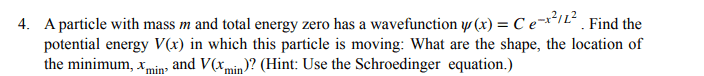# A practice problem with Schrodinger equation

drop_out_kid
Homework Statement:
In the comment section
Relevant Equations:
Schrodinger equationSo my question is.. Is schrodinger equation for this problem like this?:How to use the condition that E=0?

Thank you

Staff Emeritus
Homework Helper
The time-independent Schrödinger equation is
$$-\frac{\hbar^2}{2m}\psi''(x) + V(x)\psi(x) = E\psi(x).$$ When you set ##E=0##, the righthand side becomes 0.

•PhDeezNutz
drop_out_kid
The time-independent Schrödinger equation is
$$-\frac{\hbar^2}{2m}\psi''(x) + V(x)\psi(x) = E\psi(x).$$ When you set ##E=0##, the righthand side becomes 0.
Exactly. And I solve it as this:Could you take a look if it's right? I thought this is probably too trivial that xmin is just 0?

Staff Emeritus
Homework Helper
I'd expect there should be a constant term as well.

The given wave function is one of the energy eigenstates for the simple harmonic oscillator. What's the potential energy function for the simple harmonic oscillator?

drop_out_kid
I'd expect there should be a constant term as well.

The given wave function is one of the energy eigenstates for the simple harmonic oscillator. What's the potential energy function for the simple harmonic oscillator?
-1/2kx^2?

So where is the constant come from ?? the phi(x) got cancelled..

Thank you so much for answering my question!

Staff Emeritus
Homework Helper
##\psi''## should have two terms. Neither term disappears when you divide by ##\psi##.

drop_out_kid
##\psi''## should have two terms. Neither term disappears when you divide by ##\psi##.
Thank you so much... I am doing it again

drop_out_kid
##\psi''## should have two terms. Neither term disappears when you divide by ##\psi##.
So yes there's a constant! And I got another question: should I time a sai(t)? I am not familiar with it but I saw that phi(x,t) usually written to phi(x)*sai(t) and sai(t) is usually an exponential

LCSphysicist
So yes there's a constant! And I got another question: should I time a sai(t)? I am not familiar with it but I saw that phi(x,t) usually written to phi(x)*sai(t) and sai(t) is usually an exponential
I have no idea of what is a sai(t). But if you are solving the Schrodinger ///non-time-dependent/// equation (the one you posted (missing an E of energy) at the first post) you should not worry with time.

drop_out_kid
I have no idea of what is a sai(t). But if you are solving the Schrodinger ///non-time-dependent/// equation (the one you posted (missing an E of energy) at the first post) you should not worry with time.
Yes I think so. Now I got last problem of my assignment and last hour of due, thank you !